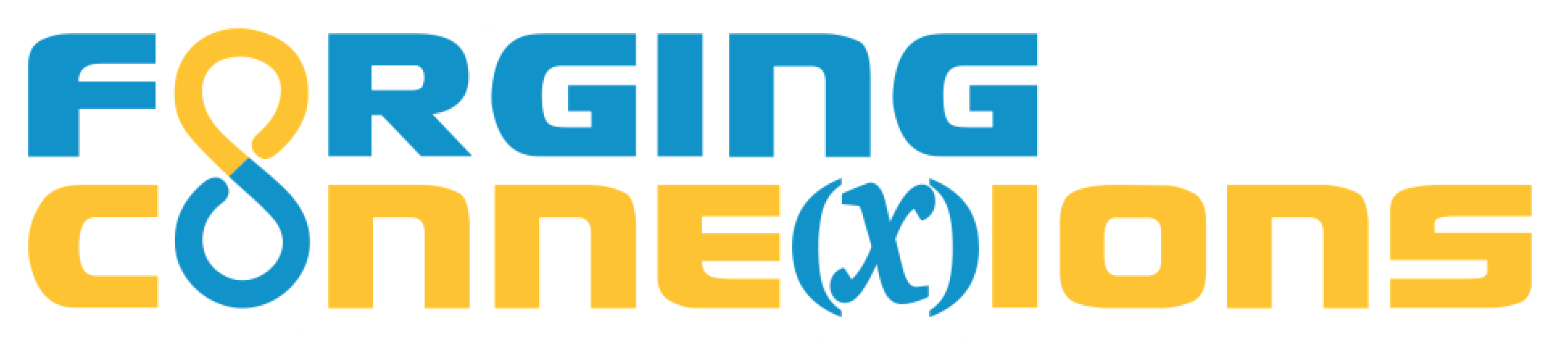Cartesian Connection

In this lesson you will use geometric transformations to construct a and you will analyze how the behavior of a function's variables determines the shape of its graph.

When you finish your construction, be sure to try the Cartesian Games. These games will challenge you while at the same time helping you to deepen your understand of functions.

1 Construct the Graph

Using the tools below, you will dilate x on a horizontal number line, rotate the dependent variable to put it on a vertical number line, and translate that result vertically.

You will use the horizontal position of x and the vertical position of T(D(x)) to construct a point with coordinates (x, T(D(x))).

By varying x and observing the resulting motion of T(D(x)) you will describe the relative motion of the two variables, and you’ll explain how the relative motion relates to the shape of the traced path of (x, T(D(x))).

Use the other pages to construct and compare functions with different values of s and v.

1. Construct a number line and make it horizontal.
2. Dilate point x on the number line.
3. Different functions can make D(x) vary faster or slower than x, and can make D(x) vary in the same or opposite direction. For each combination (faster/same, slower/same, faster/opposite, slower/opposite), find a function (using a different value of s) that produces that motion.
4. Construct a vertical number line using the same origin.
5. Transfer the value of D(x) to the vertical line.
6. How does the value of D(x) on the vertical line compare to the value of D(x) on the horizontal line? Check your result for different values of x.
7. Translate D(x) on the vertical line, attaching vector v to the vertical line.
8. Try several different functions (different values of s and v). For each function, record the values of s, v, x and T(D(x)).
9. Construct a perpendicular to the horizontal line through x. Construct a perpendicular to the vertical line through T(D(x)).
10. Drag x back and forth and observe T(D(x)).
11. What do you notice about their relative motion?
12. Trace the intersection point for different values of v.
13. What do you notice?
14. Trace the intersection point for different values of s.
15. What do you notice?
16. How does the relative speed of x and T(D(x)) affect the shape of the trace?

2 Cartesian Games: Can You Match the Mystery Function?

In this game you control a composed function TD (Translation following Dilation) by adjusting the blue dilation scale factor s and the green translation vector v. The challenge is to make your function match mystery function (shown as ??).

Directions

• When you're finished with a round, press the Reset button to reset the counters to zero and start another round.
• You can only change levels at the beginning of a new round.
• At Level 1, the values are always integers. At higher levels, they can be fractions, so you have to be more accurate at higher levels.

• Your job is to figure out the scale and vector of the mystery function ??(x). Adjust s and v to make the function you control, T(D(x)), exactly match the mystery function ??(x).
• When you have T(D(x)) exactly matching ??(x), press the Check button.
• Level 1 uses integer scale factors and vectors; higher levels use fractional values that force you to be more precise.
• On this page the two functions T\circ D and ?? are being traced. You can use the traces, or the points (x, T(D(x))) and (x, ??(x)) to observe the relative motion and adjust s and v correctly.
• On this page the two functions T\circ D and ?? are being graphed. You can use the traced graphs, or the points (x, T(D(x))) and (x, ??(x)) to observe the relative motion and adjust s and v correctly.
• On this page you have to figure out how to adjust s and v correctly without seeing the results. You will find out if your values are correct when you press the Check button.
• You’ll have to work hard to succeed on this page. If you do succeed, you’ve become a true Functionista!

3 The Fine Print

Requirements:

These activities require web access using a browser that supports HTML5 and JavaScript. (That means almost any current browser.) No purchase is required, and there’s no advertising anywhere.

Release Information

Update History:

08 Oct 2019: New version of this lesson.